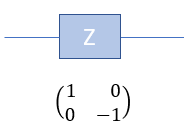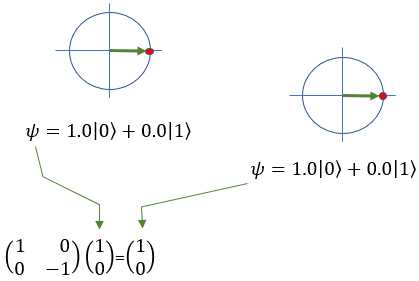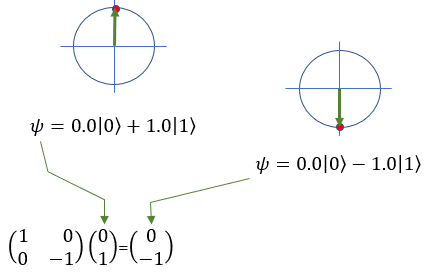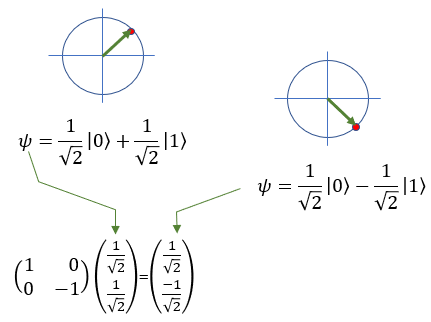Quamtum Computing                                                                        Home : www.sharetechnote.com Quamtum Gate - X (Pauli-X)   Quantom Gate X is similar to NOT gate in classical computing. As NOT gate in classical computing convert bit 0 to bit 1 and convert bit 1 to bit 0, X gate in quantum computing convert the quatum bit |0> to |1> and convert the quatum bit |1> to |0>.   In symbol and mathematical form, it is presented as follows. It takes 1 bit as input and return 1 bit as output. If you look into the matrix form, you would notice that it takes 2x1 vector (representing 1 qubit) and swap the elements within the vector.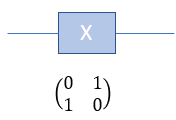If I you plug in the state vector for |0> and |1> into X gate matrix equation, you can get the output as follows. You would see that the element in the statevector get swaped by the gate X matrix.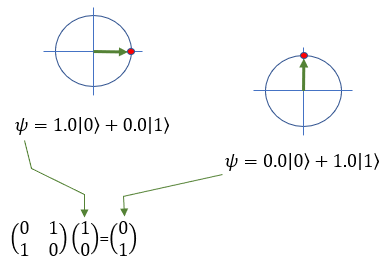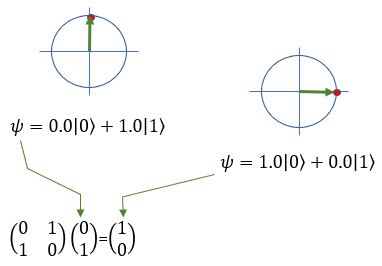Quamtum Gate - Y (Pauli-Y)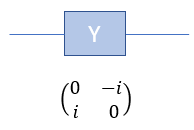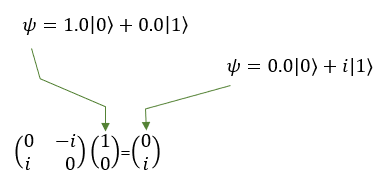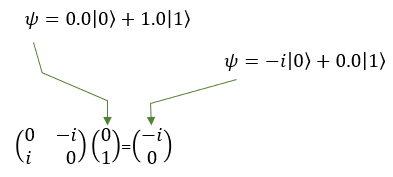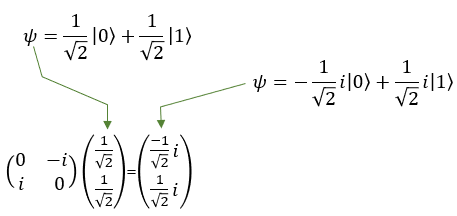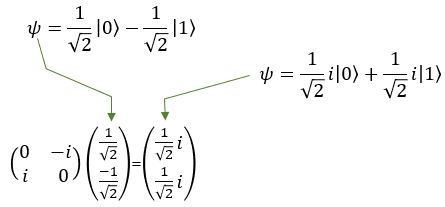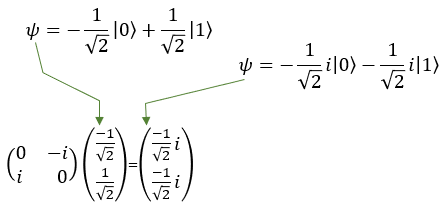Quamtum Gate - Z (Pauli-Z)